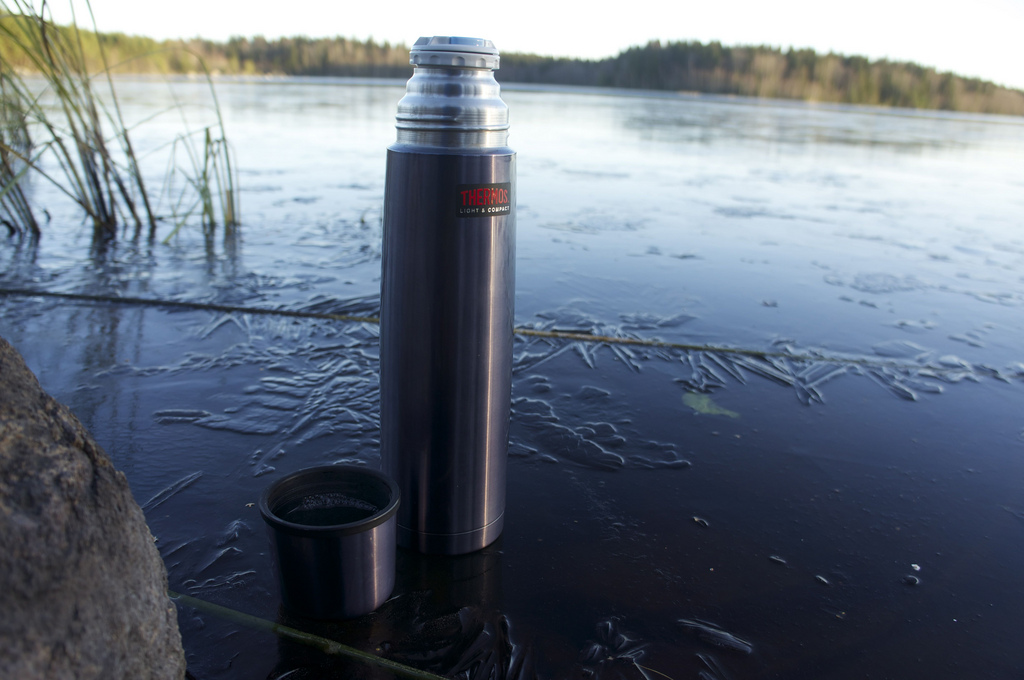# Circuitous coffee containerOver the course of a day, the outside temperature varies in a periodic fashion that can be well approximated by a sinusoid. Suppose you leave a thermos of coffee outside in the shade, measuring its temperature throughout the day, and find that it varies from 70 to 80 deg F.

Now, you make coffee in the same thermos at 200 deg F and leave it on the desk in your room. About how long (in hours) does it take for the coffee to cool to 140 deg F?

Assumptions and Details

• The outside temperature varies with time according to $T(t) = \left(75+ 15 \cos \omega t\right)\ \text{deg F}$ where $\omega = 2\pi / 24\ \text{hr}^{-1}$.
• Your room is 75 deg F.
• Your coffee follows Newton's law of cooling: $\partial_t T_\text{cup} \propto \left(T_\text{env} - T_\text{cup}\right)$.
×